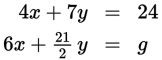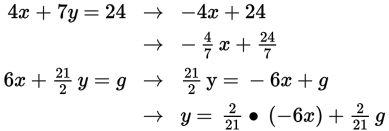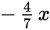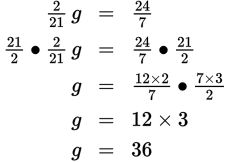# SAT Math Multiple Choice Question 807: Answer and Explanation

### Test Information

Question: 80712. In the system of equations above, g is a constant. If the system has infinitely many solutions, what is the value ofg?

• A. 16
• B. 32
• C. 36
• D. 72

Explanation:

C

Difficulty: Hard

Category: Heart of Algebra / Systems of Linear Equations

Strategic Advice: A system of equations that has infinitely many solutions indicates that the equations in the system actually represent the same line. This means you can manipulate one of the equations to look exactly like the other one.

Getting to the Answer: The easiest way to compare the equations is to rewrite them both in slope-intercept form (y = mx + b).Simplifying the x term in the second equation yields(which it should since the equations represent the same line), so all that's left to do is to set the y-intercepts (the constants) equal and solve for g: